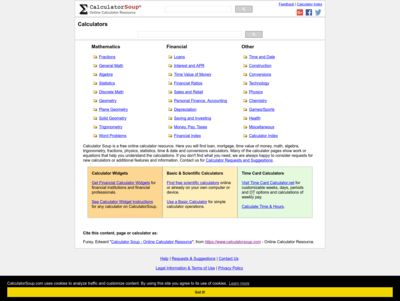Este Link não existe na sua língua, Ver em: English (en),

### https://www.calculatorsoup.com/Calculator Soup is a free online calculator resource. Here you will find loan, mortgage, time value of money, math, algebra, trigonometry, fractions, physics, statistics, time & date and conversions calculators. Many of the calculator pages show work or equations that help you understand the calculations. If you don't find what you need, we are always happy to consider requests for new calculators or additional features and information

Calculator